# Completing the square - example 1

Complete the square x^2-6x+8=0

Remember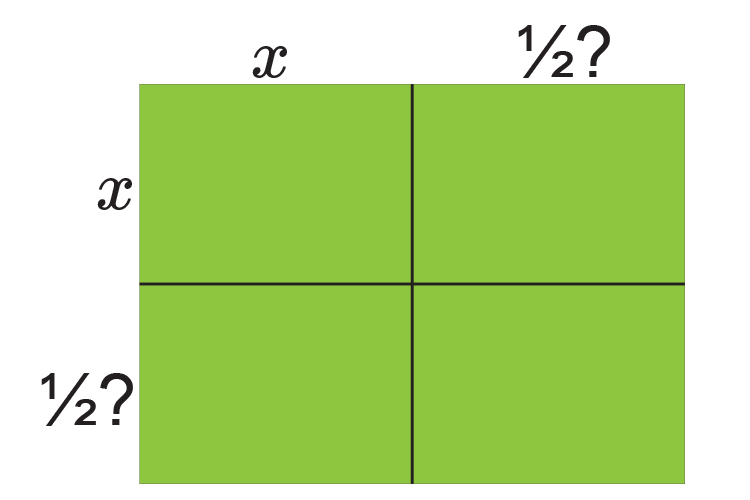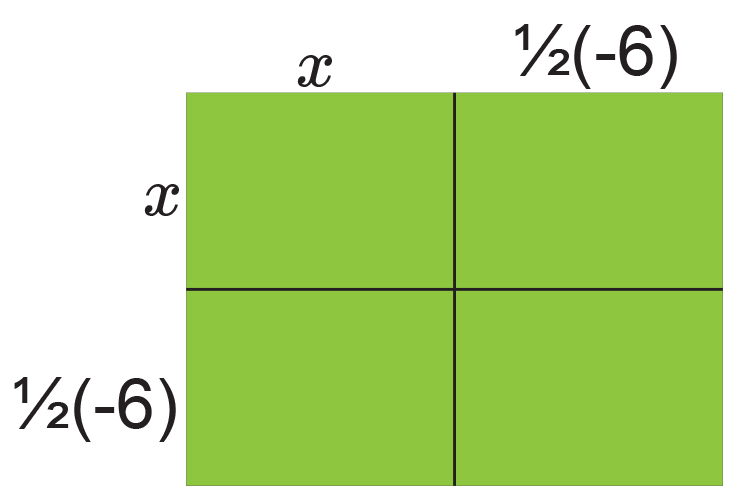Is the same as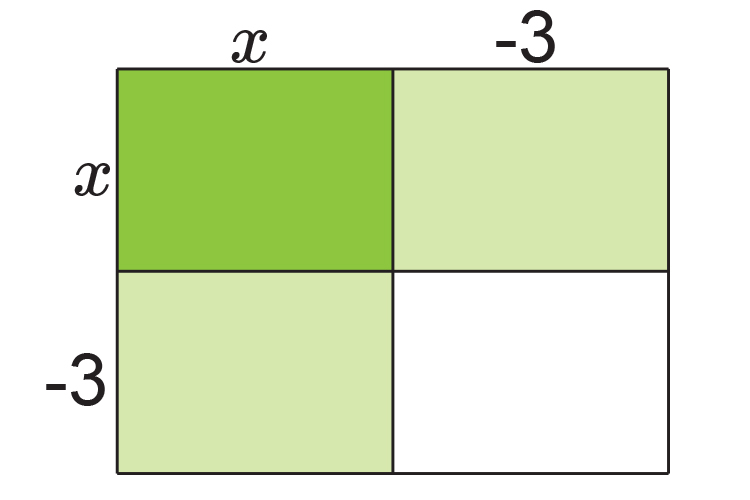Fill in the table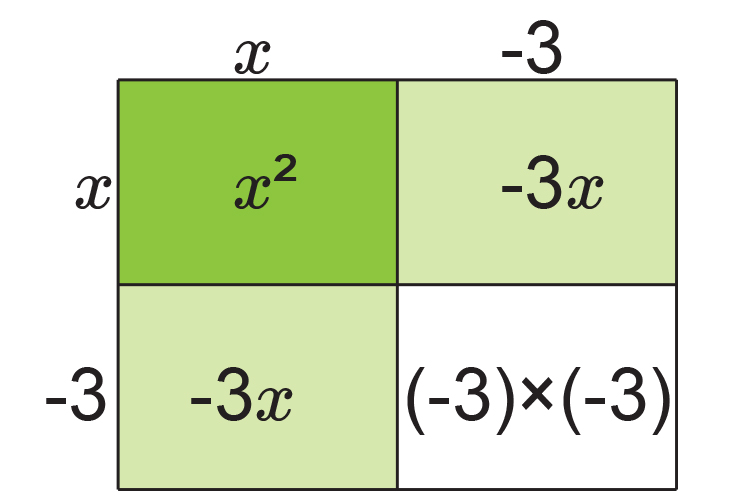Is the same as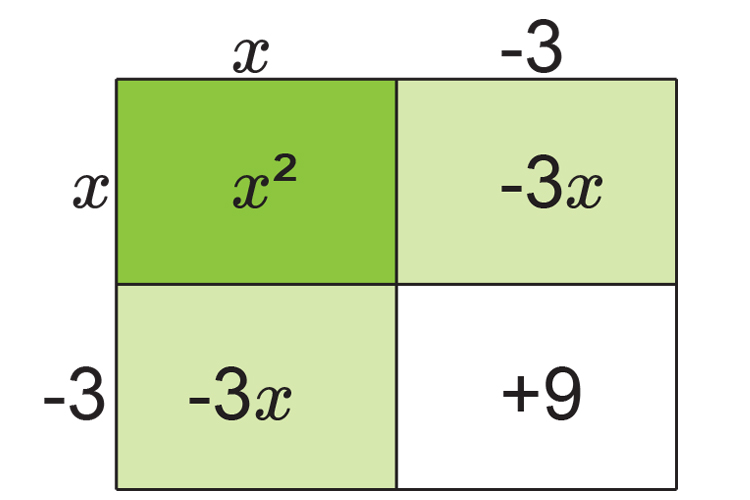NOTE:

This is the same as (x-3)^2

If you add up each area we get:

x^2-3x-3x+9

x^2-6x+9

Originally we had   x^2-6x+8=0

Now we have          x^2-6x+9=0

Always plot this on a number line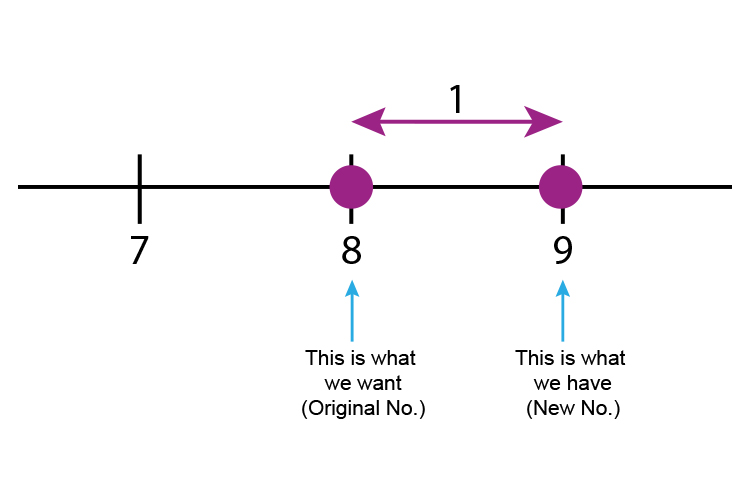The number line will help you remember original number -  new number

8-9=-1  (We need -1 )

So                        x^2-6x+8=0

Is the same as  (x-3)^2-1=0

Which can now be solved

(x-3)^2-1=0

(x-3)^2=1

x-3=+-sqrt1

(Don't forget the root of anything can be + or -)

x-3=+-1

x=3+-1

x=3+1   or   x=3-1

x=4   or   x=2

### Now check

x^2-6x+8=0

If x=4       4^2-6times4+8=0

16-24+8=0   Which is correct

If x=2       2^2-6times2+8=0

4-12+8=0     Which is correct

Answer:

The roots of x^2-6x+8=0  are x=4  and x=2